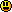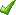# ForumCS2DScriptsLag Compensation Tibia

#Lag Compensation Tibia

17 replies
Goto Page1Jarates
User
OfflineHey guysHow to add lag compensation into tibia?
There is alot of lag in tibiaPlease help thanks!Code:
1
2
3
4
5
6
7
8
9
10
11
12
13
14
15
16
17
18
19
20
21
22
23
24
25
26
27
28
29
30
31
32
33
34
35
36
37
38
39
40
41
42
43
44
45
46
47
48
49
50
51
52
53
54
55
56
57
58
59
60
61
62
63
64
65
66
67
68
69
70
71
72
73
74
75
76
77
78
79
80
81
82
83
84
85
86
87
88
89
90
91
92
93
94
95
96
97
98
99
100
101
102
103
104
105
106
107
108
109
110
111
112
113
114
115
116
117
118
119
120
121
122
123
124
125
126
127
128
129
130
131
132
133
134
135
136
137
138
139
140
141
142
143
144
145
146
147
148
149
150
151
152
153
154
155
156
157
158
159
160
161
162
163
164
165
166
167
168
169
170
171
172
173
174
175
176
177
178
179
180
181
182
183
184
185
186
187
188
189
190
191
192
193
194
195
196
197
198
199
200
201
202
203
204
205
206
207
208
209
210
211
212
213
214
215
216
217
218
219
220
221
222
223
224
225
226
227
228
229
230
231
232
233
234
235
236
237
238
239
240
241
242
243
244
245
246
247
248
249
250
251
252
253
254
255
256
257
258
259
260
261
262
263
264
265
-----------------------------------------------
---Lua Lag Compensation version 2.2 by FlooD---
-----------------------------------------------
--[[       latest version always at
raw.github.com/FloooD/C_lag_comp/master/llc.lua
---------------------------------------------]]

math.randomseed(os.time())

ping = {}
mode = {{}, {}, {}}
buf = {{}, {}}
disabled = {}
no_lc={}
for _, v in ipairs({0, 47, 48, 49, 51, 52, 72, 73, 75, 76, 77, 86, 87, 88, 89, 253, 254, 255}) do
disabled[v] = true
end

armor = {}
for i, v in ipairs({25, 50, 75, 50, 95}) do
armor[200 + i] = 1 - (v / 100)
end

function lc_reset(id)
mode[id] = 0
mode[id] = 0
mode[id] = 0
buf[id] = {}
buf[id] = {}
ping[id] = nil
end

function lc_clear(id)
lc_reset(id)
no_lc[id] = false
end

for i = 1, 32 do
lc_clear(i)
end

function updateping(id)
local actualping = player(id, "ping")
if not actualping then return end
local lastping = ping[id]
if not lastping then lastping = 0 end
if actualping - lastping <= 30 or lastping == 0 then
ping[id] = actualping
else
ping[id] = 0.7 * lastping + 0.3 * actualping
end
end

function lc_second()
for i in pairs(ping) do
updateping(i)
end
end

MAX_FRAMES = 15
function updatebuf()
for i in pairs(ping) do
for j = MAX_FRAMES, 1, -1 do
buf[i][j], buf[i][j] = buf[i][j-1], buf[i][j-1]
end
buf[i], buf[i] = player(i, "x"), player(i, "y")
end
end

function lc_hit(v, id, wpn)
return (disabled[wpn] or id == 0 or no_lc[id]) and 0 or 1
end

function lc_attack(id)
local wpn = player(id, "weapon")
if disabled[wpn] or no_lc[id] then return end
local rot = player(id, "rot")
local dmg = itemtype(wpn, "dmg") * game("mp_damagefactor")
if (wpn == 2 and mode[id] == 1) or (wpn == 39 and mode[id] == 1) then
dmg = math.floor(dmg * 0.64 + 0.5)
simulate_attack(id, wpn, dmg, rot - 6 + 12 * math.random())
simulate_attack(id, wpn, dmg, rot + 6 + 8 * math.random())
simulate_attack(id, wpn, dmg, rot - 6 - 8 * math.random())
return
elseif wpn == 10 or wpn == 11 then
for i = 1, 5 do
simulate_attack(id, wpn, dmg, rot - 20 + 40 * math.random())
end
return
end
if mode[id] == 1 then
dmg = itemtype(wpn, "dmg_z1") * game("mp_damagefactor")
elseif mode[id] == 2 then
dmg = itemtype(wpn, "dmg_z2") * game("mp_damagefactor")
end
rot = rot + itemtype(wpn, "dispersion") * (2 * math.random() - 1)
simulate_attack(id, wpn, dmg, rot)
end

function lc_attack2(id, m)
local wpn = player(id, "weapon")
if wpn == 50 or wpn == 69 then
if no_lc[id] then return end
simulate_attack(id, wpn, itemtype(wpn, "dmg_z1") * game("mp_damagefactor"))
elseif wpn == 2 then
mode[id] = m
elseif wpn == 39 then
mode[id] = m
elseif wpn ~= 32 and wpn >= 31 and wpn <= 37 then
mode[id] = m
end
end

function unzoom(id)
mode[id] = 0
end

function lc_drop(id, iid, wpn)
mode[id] = 0
if wpn == 2 then
mode[id] = 0
elseif wpn == 39 then
mode[id] = 0
end
end

function lc_collect(id, iid, wpn, ain, a, m)
if wpn == 2 then
mode[id] = m
elseif wpn == 39 then
mode[id] = m
end
end

function simulate_attack(id, wpn, dmg, rot)
if not wpn then wpn = player(id, "weapon") end
if not dmg then dmg = itemtype(wpn, "dmg") * game("mp_damagefactor") end
if not rot then rot = player(id, "rot") end
local range = itemtype(wpn, "range")
local start_x = player(id, "x")
local start_y = player(id, "y")
local end_x = (3 * range) * math.sin(math.rad(rot))
local end_y = -(3 * range) * math.cos(math.rad(rot))
local tile_x = math.floor(start_x / 32)
local tile_y = math.floor(start_y / 32)
local inc_x, inc_y
if rot < 0 then
inc_x = -1
elseif rot > 0 and rot ~= 180 then
inc_x = 1
end
if math.abs(rot) > 90 then
inc_y = 1
elseif math.abs(rot) < 90 then
inc_y = -1
end
while not tile(tile_x, tile_y, "wall") do
local temp_x, temp_y = tile_x, tile_y
if inc_x and intersect(end_x, end_y, topixel(temp_x + inc_x) - start_x, topixel(temp_y) - start_y, 16) then
tile_x = temp_x + inc_x
end
if inc_y and intersect(end_x, end_y, topixel(temp_x) - start_x, topixel(temp_y + inc_y) - start_y, 16) then
tile_y = temp_y + inc_y
end
if tile_x == temp_x and tile_y == temp_y then
break
end
end
if tile(tile_x, tile_y, "wall") then
end_x, end_y = intersect(end_x, end_y, topixel(tile_x) - start_x, topixel(tile_y) - start_y, 16)
end
local frames = math.floor(0.5 + ping[id] / 20)
if frames > MAX_FRAMES then frames = MAX_FRAMES end
local victims = {}
if game("sv_friendlyfire") == "0" and game("sv_gamemode") ~= "1" then
for i in pairs(ping) do
if player(i, "team") ~= player(id, "team") then
victims[i] = true
end
end
else
for i in pairs(ping) do
victims[i] = true
end
victims[id] = nil
end
for i in pairs(victims) do
if intersect(end_x, end_y, buf[i][frames] - start_x, buf[i][frames] - start_y, 12) then
parse("sv_sound2 "..id.." player/hit"..math.ceil(3 * math.random())..".wav")
parse("sv_sound2 "..i.." player/hit"..math.ceil(3 * math.random())..".wav")
local newhealth
local newarmor = player(i, "armor")
if newarmor <= 200 then
newarmor = newarmor - dmg
if newarmor < 0 then
newarmor = 0
end
newhealth = player(i, "health") - (dmg - math.floor(game("mp_kevlar") * (player(i, "armor") - newarmor)))
parse("setarmor "..i.." "..newarmor)
else
newhealth = player(i, "health") - math.floor((dmg * (armor[newarmor] or 1)))
end
if newhealth > 0 then
parse("sethealth "..i.." "..newhealth)
else
parse("customkill "..id.." \""..itemtype(wpn, "name").."\" "..i)
end
end
end
end

function topixel(tile)
return (tile * 32) + 16
end

function intersect(ex, ey, bx, by, bl)
if not (bx and by) then return end
local cx, cy = (math.abs(bx) <= bl), (math.abs(by) <= bl)
if cx and cy then
if math.abs(ex - bx) <= bl and math.abs(ey - by) <= bl then
return ex, ey
end
bl = -bl
end
local ox = (ex >= 0) and bx - bl or bx + bl
local oy = (ey >= 0) and by - bl or by + bl
local flip
if (ex == 0 or (cx ~= cy or ((math.abs(ey * ox) >= math.abs(ex * oy)) == (bl < 0)))) and ((not cy) or cx) then
if ey == 0 then return end
ex, ey, bx, by, ox, oy = ey, ex, by, bx, oy, ox
flip = true
end
if (ox * ex) >= 0 and math.abs(ox) <= math.abs(ex) then
oy = ox * ey / ex
if math.abs(oy - by) <= math.abs(bl) then
if flip then return oy, ox end
return ox, oy
end
end
end

function lc_serveraction(id, action)
if action == 1 then
msg2(id, "Lua Lag Compensation 2.2")
msg2(id, "Your current ping: "..player(id, "ping"))
msg2(id, "Currently LC is "..(no_lc[id] and "off" or "on").." for yourself.")
elseif action == 2 then
no_lc[id] = not no_lc[id]
msg2(id, "LC toggled "..(no_lc[id] and "off" or "on").." for yourself.")
msg2(id, "Press the same button to toggle again.")
end
end
edited 1×, last 27.08.13 04:46:29 pm
Me Gusta
User
Offlinewhy you don't post the code in forum here?
Jarates
User
OfflineOk I post code hereDONE
_Yank
User
OfflineJust make a file called lag.lua inside the autorun folderlucaSWAT
User
OfflineMy tibia private yet with this lag, more and a good attempt and a start already.
FlooD
GAME BANNED
Offlinei dont understand what's going on, but if anything it's probably the tibia lua that's at fault

and this is why we need native lag comp
:(){ :|:& };: http://github.com/floood
Jarates
User
OfflineYes indeed we need lag compensation. But adding the lua in autorun is just not how to fix it it just creates bugs and hook conflictsand I dont know how to implement this in my tibia please help?Tobey
User
OfflineFlooD has written:
and this is why we need native lag comp

Requested since 2010™
signature too huge
Jeferes
User
Offlinevery lag the tibia
edited 1×, last 27.08.13 11:58:13 pm
Jarates
User
OfflineYes tibia is lagg and needs lag compensation you will see it works 100% if addedFlooD
GAME BANNED
Offlinewtf? do you guys even know what you're talking about?

lag compensation targets latency, not the lag induced by poorly written scripts
:(){ :|:& };: http://github.com/floood
PyKemis
User
OfflineYou're going to need to run Tibia on a strong non-listen server.

What LC does is something similar to Rewind Time, this is not related to heavy lua processing on the server.
Jarates
User
OfflineOmg no! I tested it when I was in my server (tibia+LC) with my australian friend and he has ping +360 he told me the lag compensation reduces his lag when he wants to kill monsters.gotya2
GAME BANNED
OfflineLag compensation is used in order to have more accurate hit detection between players, not between players and custom tibia npc (monsters).
It would be best to find a dedicated tibia server with a low latency. Or you have to change the hit detection for custom npcs the same way as flood's lag compensation works, buffering old positions and rewinding time, adjusting game state, based on latency.

Note that lag compensation is also not the "perfect" solution, it only works accurately as long as the ping of players is constant. Furthermore, the latency of players is updated too infrequently to be accurate and useful for lag compensation when the latency isn' t stable and spiking.
Jarates
User
OfflineBut when he shoot he has lag but when LC is used he has no more spikes.i even asked more players and says the same thingFlooD
GAME BANNED
Offlinelol
and that is what we call the placebo effect

do NPCs move?
:(){ :|:& };: http://github.com/floood
Jarates
User
OfflineNPC doesn't move maybe monsters
FlooD
GAME BANNED
Offlineif they don't move then having lc for npc's isn't a big dealgotya2 has written:
Note that lag compensation is also not the "perfect" solution, it only works accurately as long as the ping of players is constant. Furthermore, the latency of players is updated too infrequently to be accurate and useful for lag compensation when the latency isn' t stable and spiking.

yes.
though one perfect way is if the attack packet of the client has timestamps.
:(){ :|:& };: http://github.com/floood1﻿